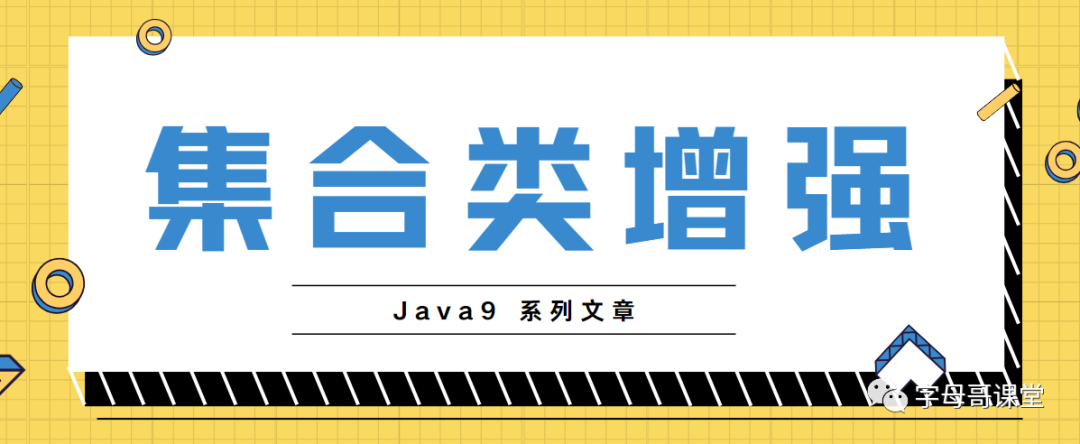# Java9系列第5篇-集合类的增强

2020/10/18 06:49## 一、提供Of()方法创建集合

### 1.1.构建Set集合对象

Set<Integer> integers = Set.of(2, 6, 7, 10);System.out.println(integers);  //[2, 10, 6, 7]

 of()//空的set of(E) of(E, E) of(E, E, E) //更多 ...... of(E, E, E, E, E, E, E, E, E, E )// 一直到十个元素 of(E...)//更多参数

### 1.2.构建List集合对象

 List<Integer> integers = List.of(2, 6, 7, 10); System.out.println(integers); // [2, 6, 7, 10]

### 1.3.构建Map对象

 Map<Integer, String> map = Map.of(2, "two", 6, "six"); System.out.println(map); // {2=two, 6=six}

### 1.4.使用Map.ofEntries() 和 Map.entry()

 Map<Integer, String> map = Map.ofEntries(Map.entry(2, "two"), Map.entry(4, "four")); System.out.println(map); //{2=two, 4=four}

## 二、Arrays

Arrays是我们进行集合操作的工具类，在Java 9 版本中也进行了增强。

### 2.1.Arrays.mismatch()

int[] ints1 = {1, 3, 5, 7, 9};int[] ints2 = {1, 3, 5, 6, 7};int i = Arrays.mismatch(ints1, ints2);System.out.println(i); //3

 int mismatch(int[] a, int aFromIndex, int aToIndex,                 int[] b, int bFromIndex, int bToIndex)

 int[] arrayA = {-2, 1, 3, 5, 7, 9}; int[] arrayB = {-1, 0, 1, 3, 5, 7, 10}; int j = Arrays.mismatch(arrayA, 1, arrayA.length, arrayB, 2, arrayB.length); System.out.println(j);

### 2.2.Arrays.equals()

Arrays.mismatch()有些相似，Arrays.equals()用来判断两个数组区间内的元素是否相等。新方法为两个被比较的数组新增了fromIndex和toIndex参数。这些方法根据两个数组的相对索引位置检查两个数组的相等性。

 String[] sa = {"d", "e", "f", "g", "h"}; String[] sb = {"a", "b", "c", "d", "e", "f"}; boolean b = Arrays.equals(sa, 0, 2, sb, 3, 5); System.out.println(b);  //true### 作者的其它热门文章

0
0 收藏

0 评论
0 收藏
0